Note: The other languages of the website are Google-translated. Back to English

## 如何将数据透视表过滤器链接到Excel中的某个单元格？

#### 使用VBA代码将数据透视表过滤器链接到特定单元格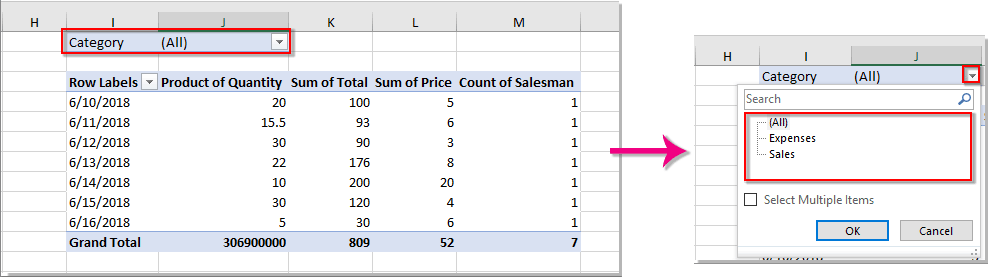1.请选择一个单元格（在这里我选择单元格H6），您将链接到数据透视表的过滤器功能，并提前在该单元格中输入一个过滤器值。

2.打开包含要链接到单元格的数据透视表的工作表。 右键单击工作表标签，然后选择 查看代码 从上下文菜单中。 看截图：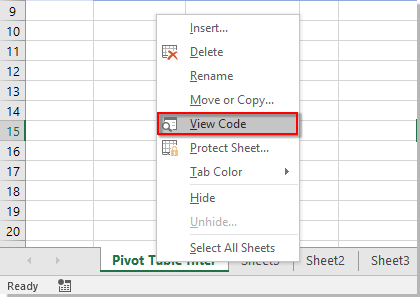3。 在里面 Microsoft Visual Basic应用程序 窗口，将下面的VBA代码复制到“代码”窗口中。

VBA代码：将数据透视表过滤器链接到某个单元格

``````Private Sub Worksheet_Change(ByVal Target As Range)
'Update by Extendoffice 20180702
Dim xPTable As PivotTable
Dim xPFile As PivotField
Dim xStr As String
On Error Resume Next
If Intersect(Target, Range("H6")) Is Nothing Then Exit Sub
Application.ScreenUpdating = False
Set xPTable = Worksheets("Sheet1").PivotTables("PivotTable2")
Set xPFile = xPTable.PivotFields("Category")
xStr = Target.Text
xPFile.ClearAllFilters
xPFile.CurrentPage = xStr
Application.ScreenUpdating = True
End Sub``````

1）“Sheet1”是打开的工作表的名称。
2）“数据透视表2”是数据透视表的名称，您会将其过滤功能链接到单元格。
3）数据透视表中的过滤字段称为“类别".
4）引用的单元格为H6。 您可以根据需要更改这些变量值。

4。 按 其他 + Q 关闭键 Microsoft Visual Basic应用程序 窗口。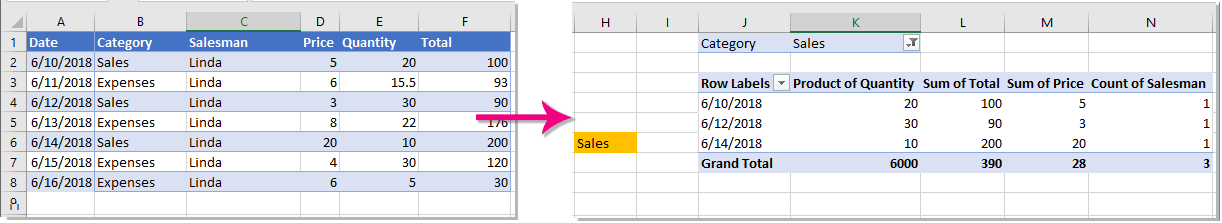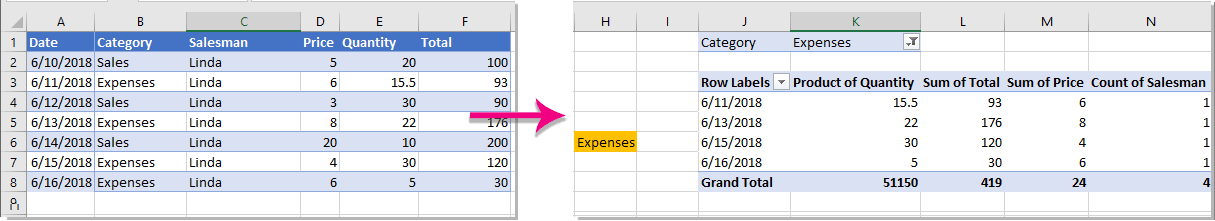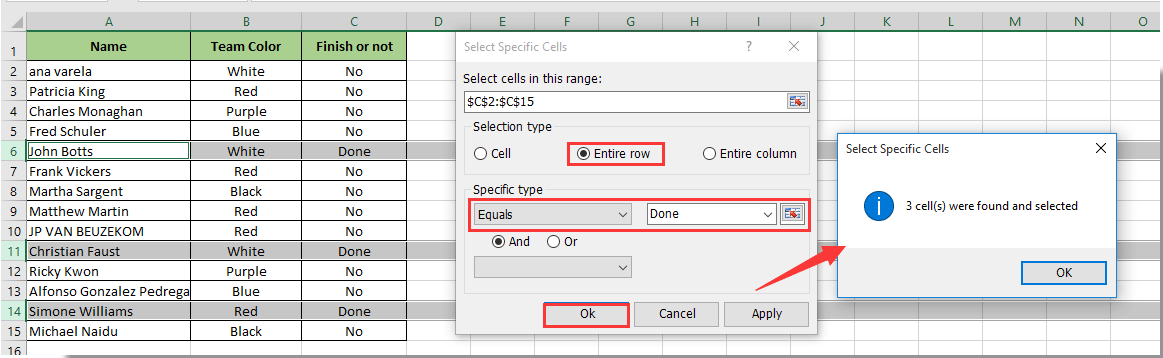### 最佳办公效率工具

#### Kutools for Excel解决了您的大多数问题，并使您的生产率提高了80％

• 重用: 快速插入 复杂的公式，图表 以及您以前使用过的任何东西； 加密单元 带密码 创建邮件列表 并发送电子邮件...
• 超级公式栏 （轻松编辑多行文本和公式）； 阅读版式 （轻松读取和编辑大量单元格）； 粘贴到过滤范围...
• 合并单元格/行/列 不会丢失数据； 拆分单元格内容； 合并重复的行/列...防止细胞重复； 比较范围...
• 选择重复或唯一 行; 选择空白行 （所有单元格都是空的）； 超级查找和模糊查找 在许多工作簿中； 随机选择...
• 确切的副本 多个单元格，无需更改公式参考； 自动创建参考 到多张纸； 插入项目符号，复选框等...
• 提取文字，添加文本，按位置删除， 删除空间; 创建和打印分页小计； 在单元格内容和注释之间转换...
• 超级滤镜 （将过滤方案保存并应用于其他工作表）； 高级排序 按月/周/日，频率及更多； 特殊过滤器 用粗体，斜体...
• 结合工作簿和工作表; 根据关键列合并表； 将数据分割成多个工作表; 批量转换xls，xlsx和PDF...
• 超过300种强大功能。 支持Office / Excel 2007-2019和365。支持所有语言。 在您的企业或组织中轻松部署。 完整功能30天免费试用。 60天退款保证。#### Office选项卡为Office带来了选项卡式界面，使您的工作更加轻松

• 在Word，Excel，PowerPoint中启用选项卡式编辑和阅读，发布者，Access，Visio和Project。
• 在同一窗口的新选项卡中而不是在新窗口中打开并创建多个文档。
• 每天将您的工作效率提高50％，并减少数百次鼠标单击！Sory 帮不了你。

H1

``````

Private Sub Worksheet_Change(ByVal Target As Range)
'Update by Extendoffice 20180702
Dim xPTable As PivotTable
Dim xPFile As PivotField
Dim xPTabled As PivotTable
Dim xPFiled As PivotField
Dim xStr As String
On Error Resume Next
'리스트 만들기
Dim listArray() As Variant
listArray = Array("PivotTable1", "PivotTable2", "PivotTable3", "PivotTable4")
If Intersect(Target, Range("H1")) Is Nothing Then Exit Sub
Application.ScreenUpdating = False
For i = 0 To UBound(listArray)
Set xPTable = Worksheets("SheetName").PivotTables(listArray(i))
Set xPFile = xPTable.PivotFields("FieldName")
'MsgBox (listArray(i))
xStr = Target.Text
xPFile.ClearAllFilters
xPFile.CurrentPage = xStr
Next
Application.ScreenUpdating = True
End Sub``````

**找到这些并更改它**

E1

'更新者 Extendoffice 20180702

'리스트 만들기

listArray = Array("数据透视表1", "数据透视表2", "数据透视表3")

Application.ScreenUpdating = False

For i = 0 To UBound(listArray)

xStr = 目标文本
xPFile.ClearAllFilters
xPFile.CurrentPage = xStr

Application.ScreenUpdating =真

END SUB

Ciao, sto provando a fare lo stesso esempio per far in modo che il filtro della pivot si setti sul valore della cella,
non riesco a farla funzionare。

Quale passaggio manca nella descrizione sopra？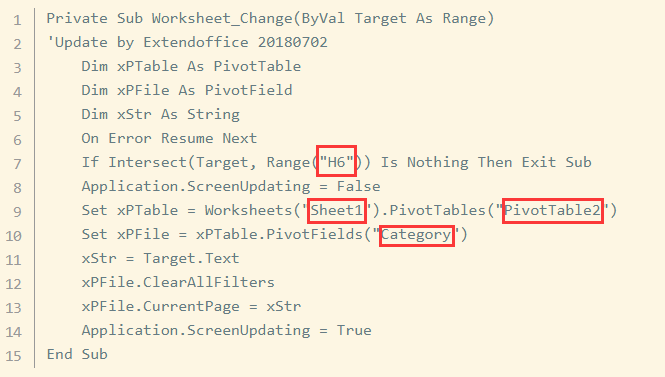'更新者 Extendoffice 20180702

Application.ScreenUpdating = False

xStr = 目标文本
xPFile.ClearAllFilters
xPFile.CurrentPage = xStr
Application.ScreenUpdating =真
END SUB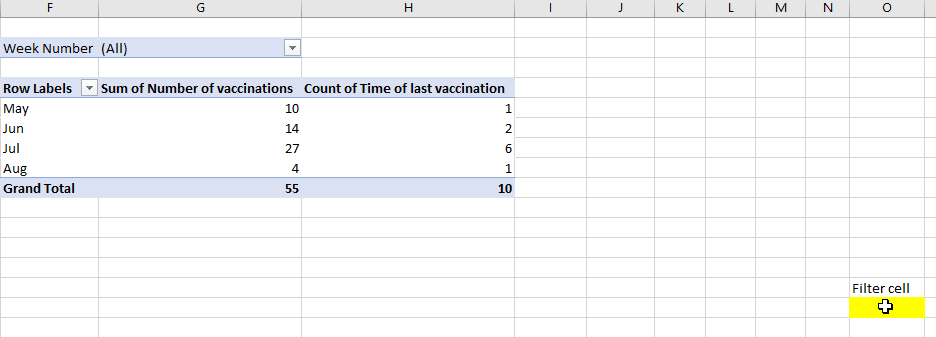``````Private Sub Worksheet_Change(ByVal Target As Range)
'Update by Extendoffice 20220706
Dim I As Integer
Dim xFilterStr1, xFilterStr2 As String
On Error Resume Next
If Intersect(Target, Range("O26:O27")) Is Nothing Then Exit Sub
'Application.ScreenUpdating = False

xFilterStr1 = Range("O26").Value
xFilterStr2 = Range("O27").Value
ActiveSheet.PivotTables("Order_Comp_B2C").PivotFields("Week Number"). _
ClearAllFilters
If xFilterStr1 = "" And xFilterStr2 = "" Then Exit Sub
ActiveSheet.PivotTables("Order_Comp_B2C").PivotFields("Week Number"). _
EnableMultiplePageItems = True
xCount = ActiveSheet.PivotTables("Order_Comp_B2C").PivotFields("Week Number").PivotItems.Count

For I = 1 To xCount
If I <> xFilterStr1 And I <> xFilterStr2 Then
ActiveSheet.PivotTables("Order_Comp_B2C").PivotFields("Week Number").PivotItems(I).Visible = False
Else
ActiveSheet.PivotTables("Order_Comp_B2C").PivotFields("Week Number").PivotItems(I).Visible = True
End If
Next

'Application.ScreenUpdating = True
End Sub``````

JAMES

xFilterStr1 = Range("O26").Value
xFilterStr2 = Range("O27").Value
yFilterstr1 = Range("p26").Value
yfilterstr2 = Range("p27").Value
ActiveSheet.PivotTables("Order_Comp_B2C_Crea").PivotFields("周数")。 _
ActiveSheet.PivotTables("Order_Comp_B2B_Crea").PivotFields("周数")。 _
ActiveSheet.PivotTables("Order_Comp_B2C_Disp").PivotFields("周数")。 _
ActiveSheet.PivotTables("Order_Comp_B2B_Disp").PivotFields("周数")。 _

ActiveSheet.PivotTables("Order_Comp_B2C_Crea").PivotFields("周数")。 _
ActiveSheet.PivotTables("Order_Comp_B2B_Crea").PivotFields("周数")。 _
ActiveSheet.PivotTables("Order_Comp_B2C_Disp").PivotFields("周数")。 _
ActiveSheet.PivotTables("Order_Comp_B2B_Disp").PivotFields("周数")。 _
EnableMultiplePageItems = True

xCount = ActiveSheet.PivotTables("Order_Comp_B2C_Crea").PivotFields("周数").PivotItems.Count
xCount = ActiveSheet.PivotTables("Order_Comp_B2B_Crea").PivotFields("周数").PivotItems.Count
yCount = ActiveSheet.PivotTables("Order_Comp_B2C_Disp").PivotFields("周数").PivotItems.Count
yCount = ActiveSheet.PivotTables("Order_Comp_B2B_Disp").PivotFields("周数").PivotItems.Count

ActiveSheet.PivotTables("Order_Comp_B2C_Crea").PivotFields("周数").PivotItems(I).Visible = False
ActiveSheet.PivotTables("Order_Comp_B2B_Crea").PivotFields("周数").PivotItems(I).Visible = False

ActiveSheet.PivotTables("Order_Comp_B2C_Crea").PivotFields("周数").PivotItems(I).Visible = True
ActiveSheet.PivotTables("Order_Comp_B2B_Crea").PivotFields("周数").PivotItems(I).Visible = True

ActiveSheet.PivotTables("Order_Comp_B2C_Disp").PivotFields("周数").PivotItems(I).Visible = False
ActiveSheet.PivotTables("Order_Comp_B2B_Disp").PivotFields("周数").PivotItems(I).Visible = False

ActiveSheet.PivotTables("Order_Comp_B2C_Disp").PivotFields("周数").PivotItems(I).Visible = True
ActiveSheet.PivotTables("Order_Comp_B2B_Disp").PivotFields("周数").PivotItems(I).Visible = True

END SUB

**找到这些并更改它**

E1

'更新者 Extendoffice 20180702

'리스트 만들기

listArray = Array("数据透视表1", "数据透视表2", "数据透视表3")

Application.ScreenUpdating = False

For i = 0 To UBound(listArray)

xStr = 目标文本
xPFile.ClearAllFilters
xPFile.CurrentPage = xStr

Application.ScreenUpdating =真

END SUB

``````Private Sub Worksheet_Change(ByVal Target As Range)
'Updated by Extendoffice 2022/07/22
Dim xPTable As PivotTable
Dim xPFile As PivotField
Dim xStr As String
Dim xBoolean As Boolean
Dim xItsRG As Range
Dim xDDs As Range
Dim xDs As Range
On Error Resume Next

xBoolean = False
Set xRg = Range("h6")

Set xItsRG = Intersect(Target, xRg)
Set xDDs = Intersect(Target.DirectDependents, xRg)
Set xDs = Intersect(Target.Dependents, xRg)
If Not (xItsRG Is Nothing) Then
xBoolean = True
ElseIf Not (xDDs Is Nothing) Then
xBoolean = True
ElseIf Not (xDs Is Nothing) Then
xBoolean = True
End If

If Not xBoolean Then Exit Sub

Application.ScreenUpdating = False
Set xPTable = Worksheets("Sheet1").PivotTables("Pivot Table 1")
Set xPFile = xPTable.PivotFields("Category")
xStr = Target.Text
xPFile.ClearAllFilters
xPFile.CurrentPage = xStr
Application.ScreenUpdating = True

End Sub
``````

``````Private Sub Worksheet_Change(ByVal Target As Range)
'Updated by Extendoffice 20220805
Dim xPTable As PivotTable
Dim xPFile As PivotField
Dim xStr As String
Dim xBoolean As Boolean
Dim xItsRG As Range
Dim xDDs As Range
Dim xDs As Range
On Error Resume Next

xBoolean = False
Set xRg = Range("D3")

Set xItsRG = Intersect(Target, xRg)
Set xDDs = Intersect(Target.DirectDependents, xRg)
Set xDs = Intersect(Target.Dependents, xRg)
If Not (xItsRG Is Nothing) Then
xBoolean = True
ElseIf Not (xDDs Is Nothing) Then
xBoolean = True
ElseIf Not (xDs Is Nothing) Then
xBoolean = True
End If

If Not xBoolean Then Exit Sub
xStr = Format(xRg.Text, "m/d/yyyy")
Application.ScreenUpdating = False
Set xPTable = Worksheets("Sheet2").PivotTables("PivotTable1")
Set xPFile = xPTable.PivotFields("Date")
xPFile.ClearAllFilters
xPFile.CurrentPage = xStr
Application.ScreenUpdating = True

End Sub``````

H1

``````Private Sub Worksheet_Change(ByVal Target As Range)
'Update by Extendoffice 20180702
Dim xPTable As PivotTable
Dim xPFile As PivotField
Dim xPTabled As PivotTable
Dim xPFiled As PivotField
Dim xStr As String
On Error Resume Next
'리스트 만들기
Dim listArray() As Variant
listArray = Array("PivotTable1", "PivotTable2", "PivotTable3", "PivotTable4")
If Intersect(Target, Range("H1")) Is Nothing Then Exit Sub
Application.ScreenUpdating = False
For i = 0 To UBound(listArray)
Set xPTable = Worksheets("SheetName").PivotTables(listArray(i))
Set xPFile = xPTable.PivotFields("FieldName")
'MsgBox (listArray(i))
xStr = Target.Text
xPFile.ClearAllFilters
xPFile.CurrentPage = xStr
Next
Application.ScreenUpdating = True
End Sub``````

0   产品特性